# Which one of the compounds below has the highest solubility in water, expressed in moles per liter? a. ZnCO3, Ksp = 1.4 × 10–11 b. Cd(OH)2, Ksp = 2.4 × 10–14 c. AgI, Ksp = 8.3 × 10–17 d. CdCO3, Ksp = 5.2 × 10–12

Question
28 views

Which one of the compounds below has the highest solubility in water, expressed in moles per liter? a. ZnCO3, Ksp = 1.4 × 10–11 b. Cd(OH)2, Ksp = 2.4 × 10–14 c. AgI, Ksp = 8.3 × 10–17 d. CdCO3, Ksp = 5.2 × 10–12

check_circle

Introduction:

On dissolving any component in solution, results the establishment of equilibrium among dissociated and non-dissociated ions. This solubility or dissociated ion amount is estimated by its solubility product. If Ksp is high then the compound is highly soluble in the solution.

Given: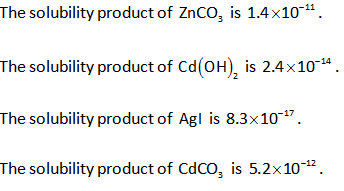Calculations and Explanation:

Zinc carbonate dissociates into ions as shown below.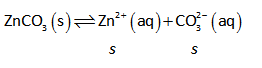Here,

The solubility of zinc carbonate is “s”.

The solubility of zinc carbonate is calculated as follows,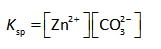Substitute the known values in the formula.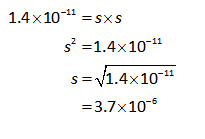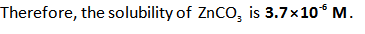Cadmium hydroxide dissociates in ions as shown below.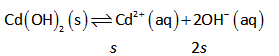Similarly, the solubility of cadmium hydroxide is calculated as follows,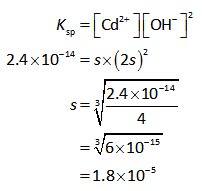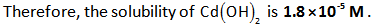The dissociation of silver iodide is shown below.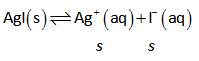The solubility of AgI is calculated as follows,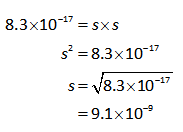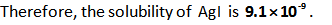Cadmium carbonate dissociates as shown below.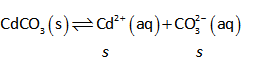The solubility of cadmium carbonate is calculated as follows,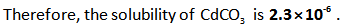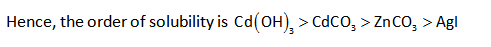...

### Want to see the full answer?

See Solution

#### Want to see this answer and more?

Solutions are written by subject experts who are available 24/7. Questions are typically answered within 1 hour.*

See Solution
*Response times may vary by subject and question.
Tagged in